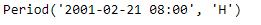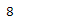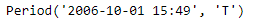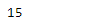Open in App
Not now

# Python | Pandas Period.hour

• Last Updated : 19 Aug, 2021

Python is a great language for doing data analysis, primarily because of the fantastic ecosystem of data-centric python packages. Pandas is one of those packages and makes importing and analyzing data much easier.

Pandas Period.hour attribute returns an integer value indicating the value of the hour in the given Period object.

Syntax : Period.hour
Parameters : None
Return : hour value

Example #1: Use Period.hour attribute to find the value of the hour in the given Period object.

## Python3

 `# importing pandas as pd``import` `pandas as pd` `# Create the Period object``prd ``=` `pd.Period(freq ``=``'H'``, year ``=` `2001``, month ``=` `2``, day ``=` `21``, hour ``=` `8``)` `# Print the Period object``print``(prd)`

Output :Now we will use the Period.hour attribute to find the hour value in the prd object.

## Python3

 `# return the hour value``prd.hour`

Output :As we can see in the output, the Period.hour attribute has returned ‘8’ indicating that the hour value in the following object is 8.

Example #2: Use Period.hour attribute to find the value of the hour in the given Period object.

## Python3

 `# importing pandas as pd``import` `pandas as pd` `# Create the Period object``prd ``=` `pd.Period(freq ``=``'T'``, year ``=` `2006``, month ``=` `10``, hour ``=` `15``, minute ``=` `49``)` `# Print the Period object``print``(prd)`

Output :Now we will use the Period.hour attribute to find the hour value in the prd object.

## Python3

 `# return the hour value``prd.hour`

Output :As we can see in the output, the Period.hour attribute has returned ’15’ indicating that the hour value in the following object is 15.

My Personal Notes arrow_drop_up